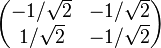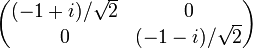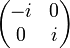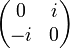# Faithful irreducible representation of dihedral group:D16

## Representation table

There are two such representations, and they are related by the group automorphism$a \mapsto a^3, x \mapsto x$, and also by the Galois automorphism$\sqrt{2} \mapsto -\sqrt{2}$ for the extension$\mathbb{Q}(\sqrt{2})$ over$\mathbb{Q}$.

We give each of these representations in three forms. One is a representation as orthogonal matrices (with the generator$a$ mapping to rotation by an odd multiple of$\pi/4$ and$x$ mapping to a reflection), and this representation is realized over the ring$\mathbb{Z}[1/\sqrt{2}]$. The second is as complex unitary matrices. The third epresentation is realized over the smaller subring$\mathbb{Z}[\sqrt{2}]$ but the matrices are no longer orthogonal matrices.

Here is the first representation in all three forms:

The table below is incomplete, it has only 11 of the 16 elements, more will be added later

Element Matrix as real orthogonal Matrix as complex unitary Matrix as real, non-orthogonal, in$\mathbb{Z}[\sqrt{2}]$ Characteristic polynomial Minimal polynomial Trace, character value Determinant$e$$\begin{pmatrix} 1 & 0 \\ 0 & 1 \\\end{pmatrix}$$\begin{pmatrix} 1 & 0 \\ 0 & 1 \\\end{pmatrix}$$\begin{pmatrix} 1 & 0 \\ 0 & 1 \\\end{pmatrix}$$(t - 1)^2 = t^2 - 2t + 1$$t - 1$ 2 1$a$$\begin{pmatrix} 1/\sqrt{2} & -1/\sqrt{2} \\ 1/\sqrt{2} & 1/\sqrt{2} \\\end{pmatrix}$$\begin{pmatrix} (1 + i)/\sqrt{2} & 0 \\ 0 & (1 - i)/\sqrt{2}\\\end{pmatrix}$$\begin{pmatrix} 0 & -1 \\ 1 & \sqrt{2} \\\end{pmatrix}$$t^2 - \sqrt{2} t + 1$$t^2 - \sqrt{2} t + 1$$\sqrt{2}$ 1$a^2$$\begin{pmatrix} 0 & -1 \\ 1 & 0 \\\end{pmatrix}$$\begin{pmatrix} i & 0 \\ 0 & -i \\\end{pmatrix}$$\begin{pmatrix} -1 & -\sqrt{2} \\ \sqrt{2} & 1 \\\end{pmatrix}$$t^2 + 1$$t^2 + 1$ 0 1$a^3$$\begin{pmatrix} -1/\sqrt{2} & -1/\sqrt{2} \\ 1/\sqrt{2} & -1/\sqrt{2} \\\end{pmatrix}$$\begin{pmatrix} (-1 + i)/\sqrt{2} & 0 \\ 0 & (-1-i)/\sqrt{2}\\\end{pmatrix}$$\begin{pmatrix} -\sqrt{2} & -1 \\ 1 & 0 \\\end{pmatrix}$$t^2 + \sqrt{2}t + 1$$t^2 + \sqrt{2}t + 1$$-\sqrt{2}$ 1$a^4$$\begin{pmatrix} -1 & 0 \\ 0 & -1 \\\end{pmatrix}$$\begin{pmatrix} -1 & 0 \\ 0 & -1 \\\end{pmatrix}$$\begin{pmatrix} -1 & 0 \\ 0 & -1 \\\end{pmatrix}$$(t + 1)^2 = t^2 + 2t + 1$$t + 1$ -2 1$a^5$$\begin{pmatrix} -1/\sqrt{2} & 1/\sqrt{2} \\ -1/\sqrt{2} & -1/\sqrt{2} \\\end{pmatrix}$$\begin{pmatrix} (-1 - i)/\sqrt{2} & 0 \\ 0 & (-1 + i)/\sqrt{2} \\\end{pmatrix}$$\begin{pmatrix} 0 & 1 \\ -1 & -\sqrt{2} \\\end{pmatrix}$$t^2 + \sqrt{2}t + 1$$t^2 + \sqrt{2}t + 1$$-\sqrt{2}$ 1$a^6$$\begin{pmatrix} 0 & 1 \\ -1 & 0 \\\end{pmatrix}$$\begin{pmatrix} -i & 0 \\ 0 & i \\\end{pmatrix}$$\begin{pmatrix} 1 & \sqrt{2} \\ -\sqrt{2} & -1 \\\end{pmatrix}$$t^2 + 1$$t^2 + 1$ 0 1$a^7$$\begin{pmatrix} 1/\sqrt{2} & 1/\sqrt{2} \\ -1/\sqrt{2} & 1/\sqrt{2} \\\end{pmatrix}$$\begin{pmatrix} (1 - i)/\sqrt{2} & 0 \\ 0 & (1 + i)/\sqrt{2}\\\end{pmatrix}$$\begin{pmatrix} \sqrt{2} & 1 \\ -1 & 0 \\\end{pmatrix}$$t^2 - \sqrt{2}t + 1$$t^2 - \sqrt{2}t + 1$$\sqrt{2}$ 1$x$$\begin{pmatrix} 1 & 0 \\ 0 & -1 \end{pmatrix}$$\begin{pmatrix} 0 & 1 \\ 1 & 0 \\\end{pmatrix}$$\begin{pmatrix}0 & 1 \\ 1 & 0 \\\end{pmatrix}$$t^2 - 1$$t^2 - 1$ 0 -1$ax$$\begin{pmatrix} 1/\sqrt{2} & 1/\sqrt{2} \\ 1/\sqrt{2} & -1/\sqrt{2} \\\end{pmatrix}$$\begin{pmatrix} 0 & (1 + i)/\sqrt{2} \\ (1 - i)/\sqrt{2} & 0 \\\end{pmatrix}$$\begin{pmatrix} -1 & 0 \\ \sqrt{2} & 1 \\\end{pmatrix}$$t^2 - 1$$t^2 - 1$ 0 -1$a^2x$$\begin{pmatrix} 0 & 1 \\ 1 & 0 \\\end{pmatrix}$$\begin{pmatrix}0 & i \\ -i & 0 \\\end{pmatrix}$$\begin{pmatrix} -\sqrt{2} & -1 \\ 1 & \sqrt{2} \\\end{pmatrix}$$t^2 - 1$$t^2 - 1$ 0 -1